Get instant live expert help with Excel or Google Sheets“My Excelchat expert helped me in less than 20 minutes, saving me what would have been 5 hours of work!”

#### Post your problem and you’ll get expert help in seconds.

Your message must be at least 40 characters
Our professional experts are available now. Your privacy is guaranteed.

# Break ties with helper column and COUNTIF

We can break ties (the same values) in a data with the COUNTIF function and a helper column. This effectively removes duplicates and removes confusion about the data. The steps below will guide all levels of excel users through the process.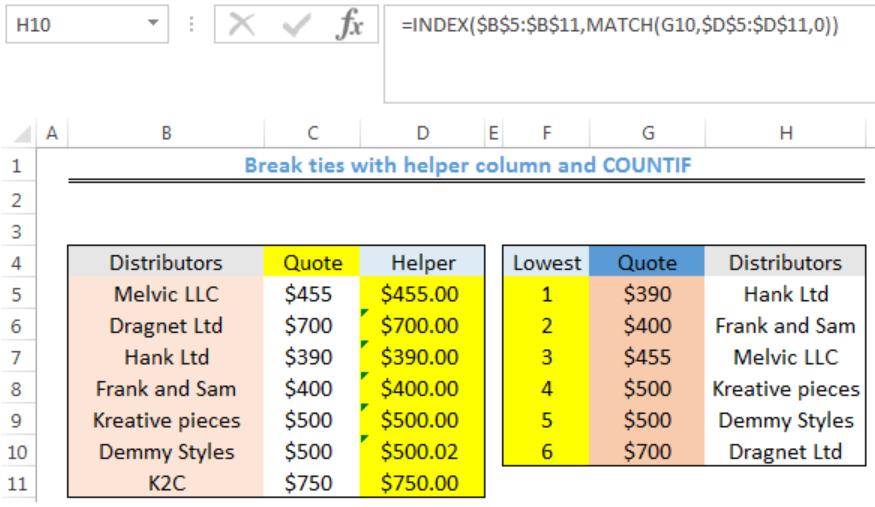Figure 1: How to Break ties with helper column and COUNTIF

## General Formula

`=A1+(COUNTIF(exp_rng,A1)-1)*adjustment`

## Formulas

Helper Column: `=C5+(COUNTIF(\$C\$5:C5,C5)-1)*0.02`

Quote: `=SMALL(\$D\$5:\$D\$11,F5)`

Distributors: `=INDEX(\$B\$5:\$B\$11,MATCH(G5,\$D\$5:\$D\$11,0))`

## Setting up the Data

• We will set up the data by inputting the Distributors and their Quotes in Column B and Column C respectively
• After generating the values for the helper column, we will rank the quotes of the distributors to get the 6 lowest quotes.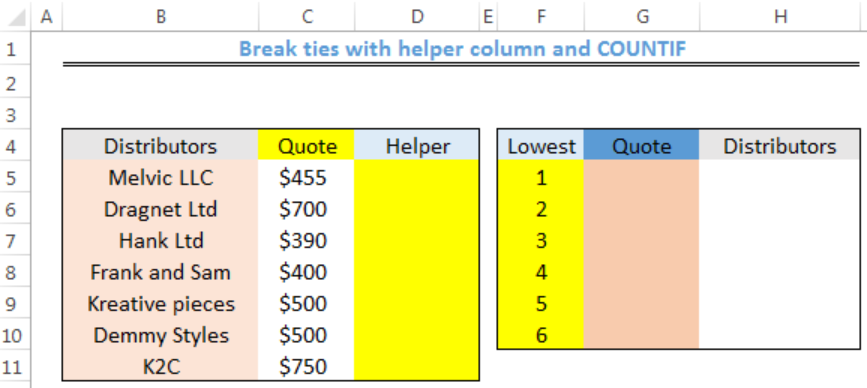Figure 2: Setting up the Data

## Generating the Helper Column

• We will click on Cell D5
• We will insert the formula below into the cell
`=C5+(COUNTIF(\$C\$5:C5,C5)-1)*0.02`
• We will press the enter key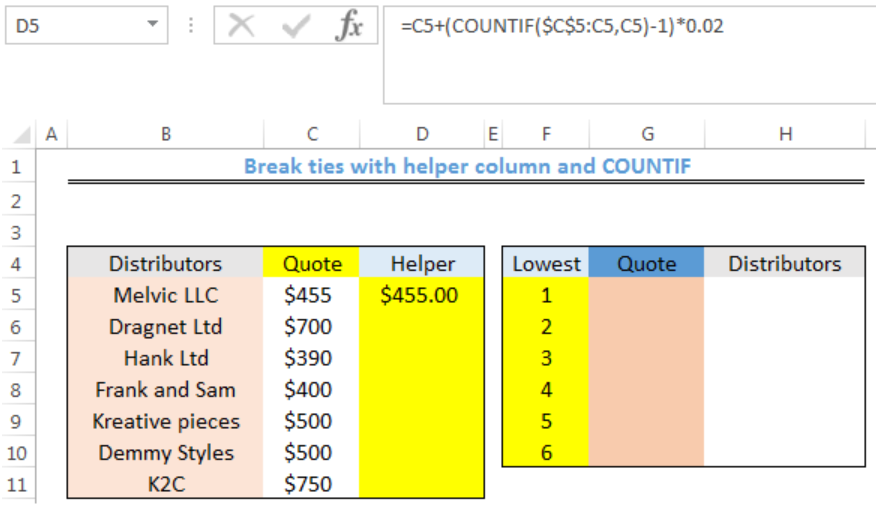Figure 3: Generating the Helper Column

• We will click on Cell D5 again
• We will double click on the fill handle tool which is the small plus sign you see at the bottom right of Cell D5. Select and drag down to copy the formula to other cells.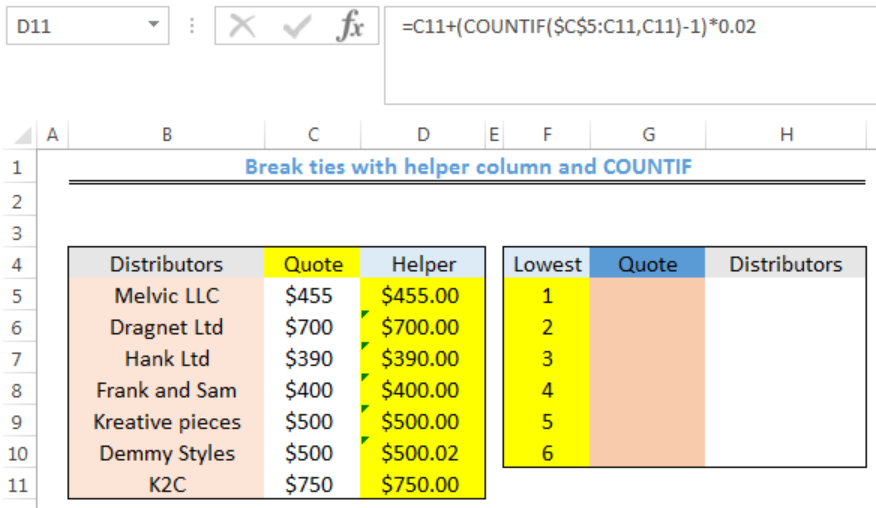Figure 4: Helper Column Generated

• We will notice that the quotes of Kreative pieces and Demmy Styles have been distinguished with the helper column

## Getting the Quotes

• We will click on Cell G5
• We will insert the formula below into the cell
`=SMALL(\$D\$5:\$D\$11,F5)`
• We will press the enter key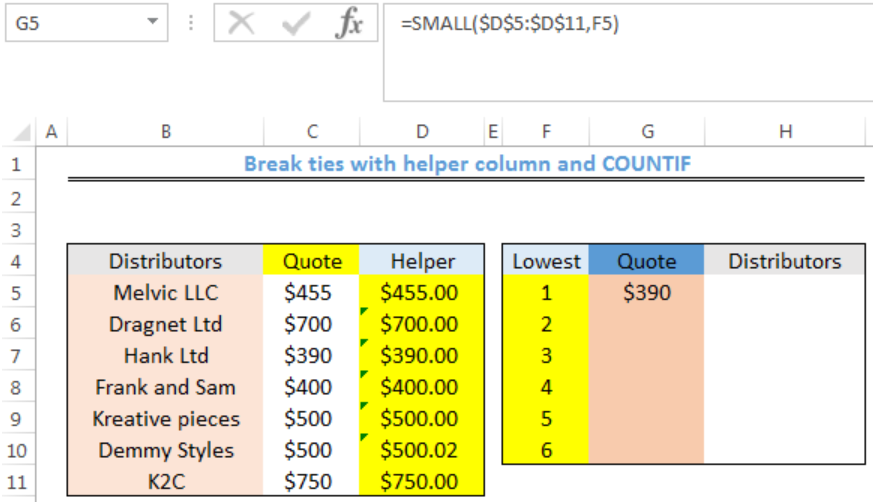Figure 5: Getting the Quotes

• We will click on Cell G5 again
• We will double click on the fill handle tool which is the small plus sign you see at the bottom right of Cell G5. Select and drag down to copy the formula to other cells.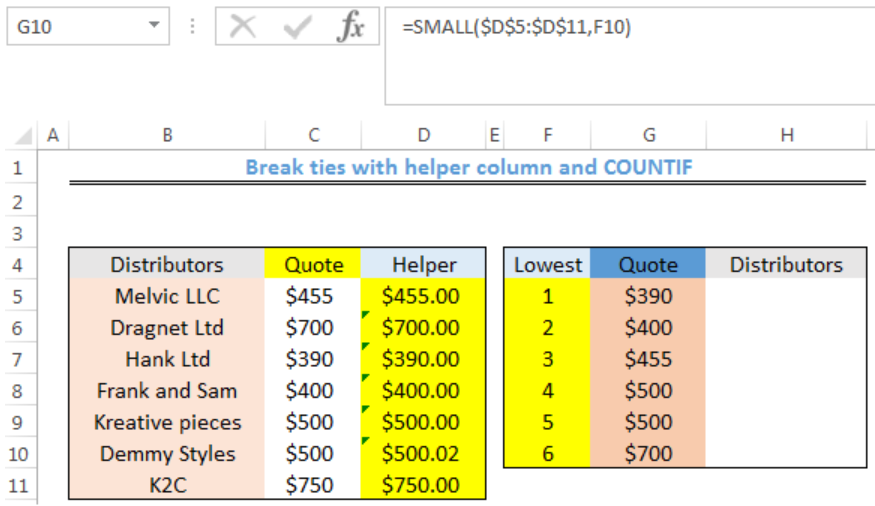Figure 6: Generated Quotes

## Getting the Distributors

• We will click on Cell H5
• We will insert the formula below into the cell
`=INDEX(\$B\$5:\$B\$11,MATCH(G5,\$D\$5:\$D\$11,0))`
• We will press the enter key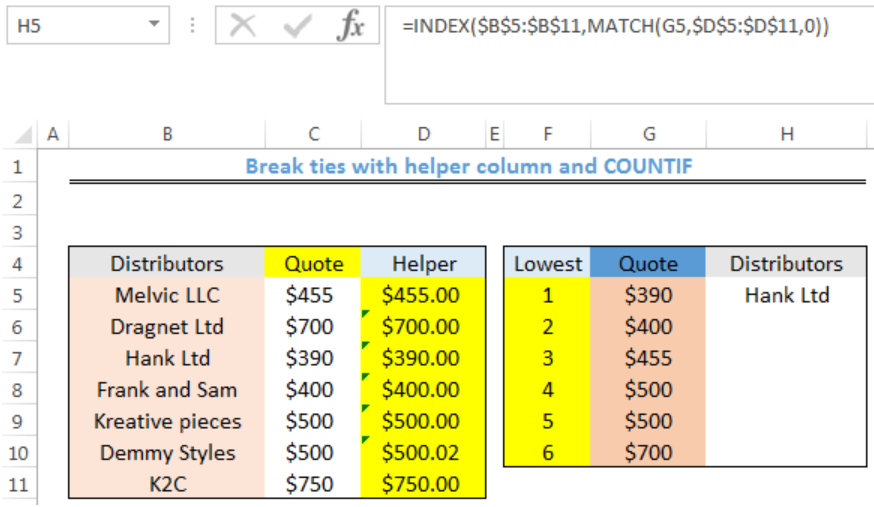Figure 7: Getting the Distributors

• We will click on Cell H5 again
• We will double click on the fill handle tool which is the small plus sign you see at the bottom right of Cell H5. Select and drag down to copy the formula to other cells.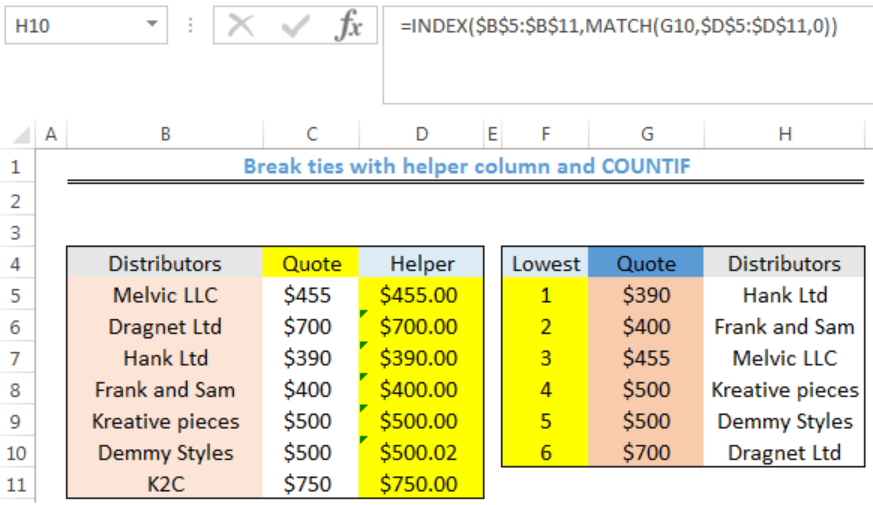Figure 8: Distributors

## Explanation

`=C5+(COUNTIF(\$C\$5:C5,C5)-1)*0.02`

If we had ranked the quote with the SMALL FUNCTION without the helper column, we will have two ranks with \$500 and we won’t know the distributors the quotes belong to. The helper column sorts this out by leaving the first tie value as it is and uses the multiplier (0.02) from the formula to rank the second tie value.

COUNTIF function utilizes an expanding range to return a running count of occurrences, rather than a total count for each value.

`=COUNTIF(\$C\$5:C5,C5)`

1 is subtracted from the result and all non-duplicate values will be zero. The result is multiplied by 0.02. This value is the “adjustment.” The adjustment should be kept small so that we do not impact the original value.

## Instant Connection to an Expert through our Excelchat Service

Most of the time, the problem you will need to solve will be more complex than a simple application of a formula or function. If you want to save hours of research and frustration, try our live Excelchat service! Our Excel Experts are available 24/7 to answer any Excel question you may have. We guarantee a connection within 30 seconds and a customized solution within 20 minutes.

### Did this post not answer your question? Get a solution from connecting with the expert.Another blog reader asked this question today on Excelchat:
Solution examplesI need a formula to count the number of rows that contain at least one of two possible phrases, but not give me the total number of instances that both phrases occur overall.
Solved by E. B. in 33 minsi have a table with Dates, First names , Last names and i want to count how many times a name occurs, but if a name occurs more than once on a particular date i need to only count it once. this is on excel and not google sheets
Solved by F. H. in 31 minsNeed a formula that counts (1,2,3,4 ect..in cell M21) a range of cells, N4:N20, which are annual premium values. But I only want to add a sale count if the average monthly premium is above \$30 per sale. So if someone has 4 sales but they don't equal a total of \$120/mth (\$30 x 4 = \$120)... they would only get a count of 3 sales.
Solved by S. E. in 60 minsHello, I am trying to create a total color column? Can anyone help?
Solved by I. A. in 60 minsAny rep that has orders over 500 and occurrences at zero for each month, for a 6 month period. I need a sum
Solved by C. E. in 60 mins## Subscribe to Excelchat.coAnother blog reader asked this question today on Excelchat: